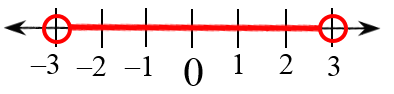### Home > INT3 > Chapter 6 > Lesson 6.1.2 > Problem6-21

6-21.

Solve each inequality and graph its solution on a number line.

See the Math Notes box in Lesson 4.2.2.

1. $|x|<3$

$−31. $|2x+1|<3$

1. $|2x+1|\ge3$